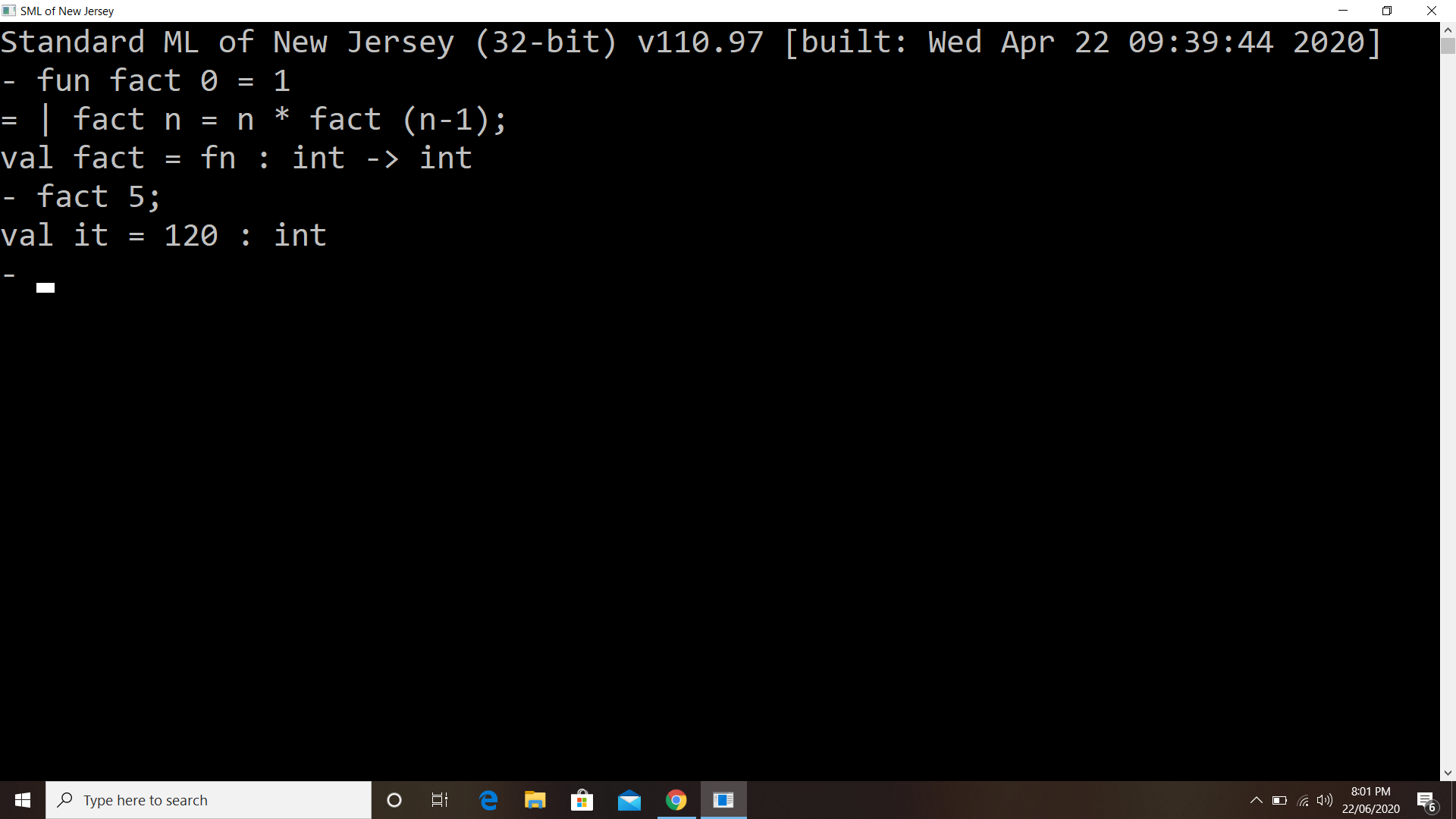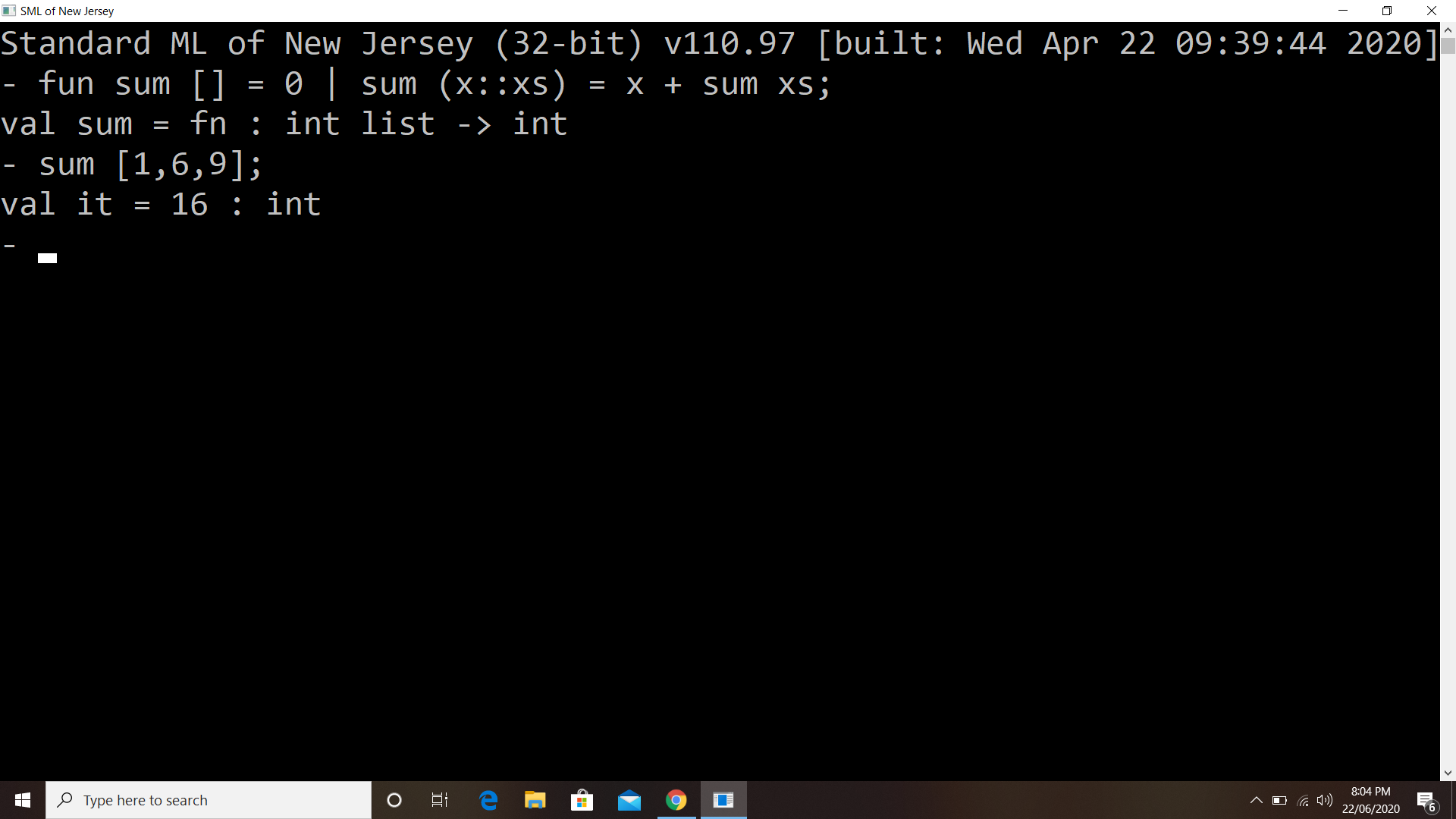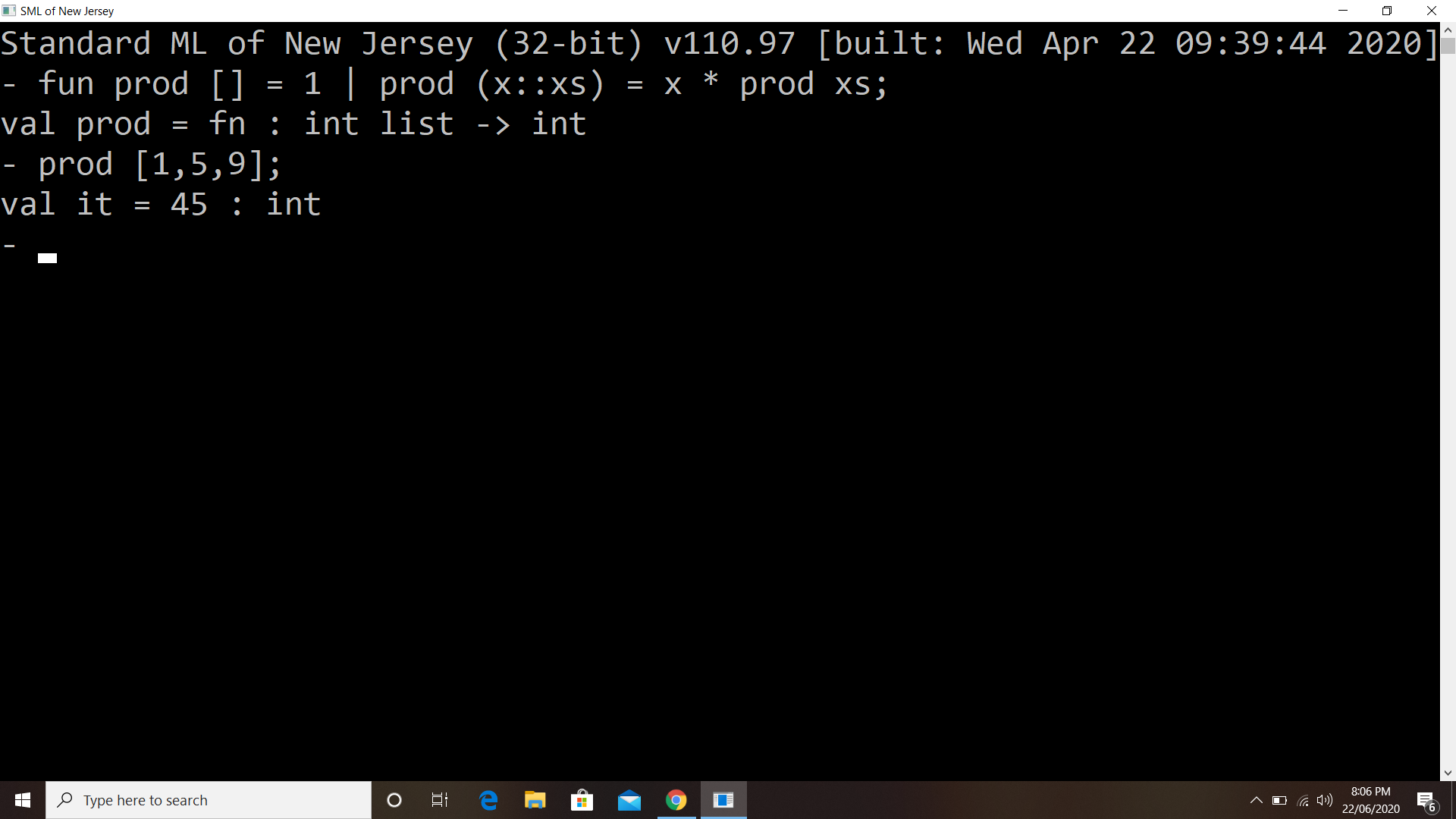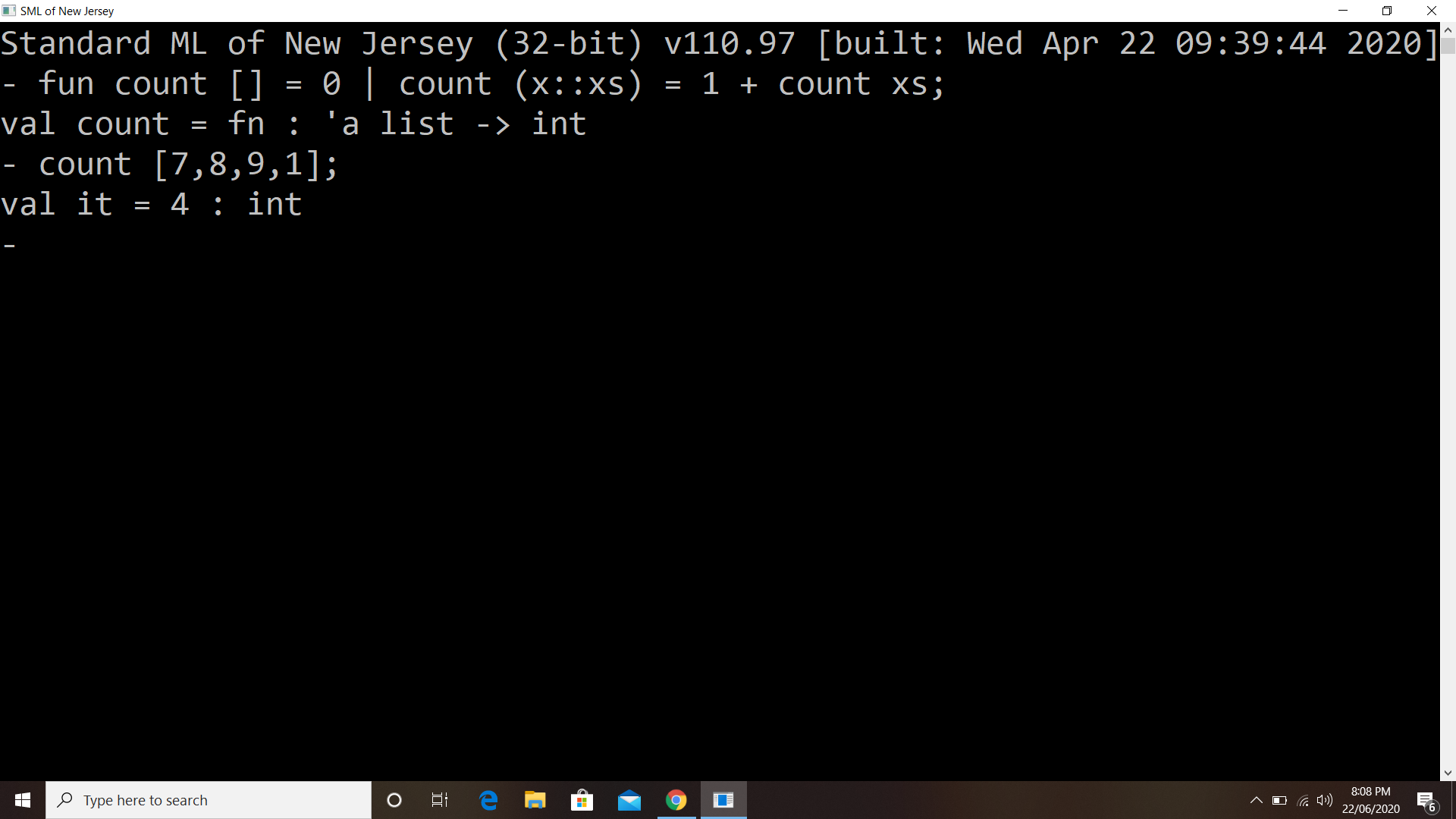Open in App
Not now

# What is Standard Meta Language (SML)?

• Difficulty Level : Basic
• Last Updated : 18 Mar, 2023

Standard Meta Language (SML) is a type-safe programming language that encapsulates numerous innovative ideas in programming language plan or design. It is a statically composed language, with an extensible type framework. It supports polymorphic type inference, which eliminates the burden of specifying types of variables and significantly encourages code re-use. It provides a productive programmed capacity for data structures and functions. It is a general-purpose, particular, functional programming language with compile-type checking and type inference. It is mainstream among compiler scholars and programming language analysts, as well as in the improvement of proving theorems. It is an advanced version of ML, the programming language utilized in the Logic for Computable Functions (LCF) hypothesis-demonstrating project. It is particular among generally utilized languages that have a proper specification, given as typing rules and operational semantics which was fully developed in 1997.

### Basic Elements of SML

1. Expression: An expression is represented using the operands and operators. In this, Infix expression is required for the evaluation of expressions.
Example:

```- 10 + 20
val it = 30 : int```

2. Variables: Here, the variables can be defined using the val keyword
Example:

```- val a = 2;
- val b = 3;
- val c = a + b;
val c = 5 : int
-val negvar=~3; (* negetive value in SML *)
val negvar = ~3```

3. Print: The print command is used to display the output in SML.
Example:

```- print ("Hello this is my first program in SML ");
Hello this is my first program in SML val it = () : unit```

4. Conditional Statements: The condition can be specified using if-else keywords.
Example:

```- if 10 > 20 then print ("Yes") else print ("No");
No val it = () : unit```

5. Functions or Procedure: The operator fun is used to define the functions.
Example:

`- fun fact 0 = 1`

6. Recursion: A recursion is an approach in which function calls itself within an argument and upon reaching the termination condition the control returns to the calling function. There are two properties that a recursive function must have:

1. Base Criteria: There must be at least one base criteria or condition such that when this condition is met the function stops calling itself recursively.
2. Progressive Approach: The recursive calls should progress in such a way that each time a recursive call is made it comes closer to the base criteria. Many programming languages implement recursion using the stack data structure.

Example of recursion in a factorial program developed in SML7. List manipulation and List processing in SML: List is represented as:

Example:

```- [10, 20, 30, 40];
val it = [10, 20, 30, 40] : int list```

### Examples

Example#1: To find the sum of all the elements of a list.Example#2: To find the product of all the elements of a list.Example#3: To find the length of a list without using length keyword.### Functions in SML

```(* this function correct only for y >= 0 *)
fun pow (x:int, y:int) =
if y=0
then 1
else x * pow(x,y-1)
fun cube (x:int) =
pow(x,3)
val sixtyfour = cube(4)```

### Functions on Lists

```(* Functions taking or producing lists *)
fun sum_list (xs : int list) =
if null xs
then 0
else hd(xs) + sum_list(tl(xs))
fun countdown (x : int) =
if x=0
then []
else x :: countdown(x-1)
fun append (xs : int list, ys : int list) = (* part of the course logo :) *)
if null xs
then ys
else hd(xs) :: append(tl(xs), ys)
(* More functions over lists, here lists of pairs of ints *)
fun sum_pair_list (xs : (int * int) list) =
if null xs
then 0
else #1 (hd(xs)) + #2 (hd(xs)) + sum_pair_list(tl(xs))
fun firsts (xs : (int * int) list) =
if null xs
then []
else (#1 (hd xs))::(firsts(tl xs))
fun seconds (xs : (int * int) list) =
if null xs
then []
else (#2 (hd xs))::(seconds(tl xs))
fun sum_pair_list2 (xs : (int * int) list) =
(sum_list (firsts xs)) + (sum_list (seconds xs))```

### Let Expressions in SML :

```(* badly named: evaluates to 0 on empty list *)
fun bad_max (xs : int list) =
if null xs
then 0
else if null (tl xs)
then hd xs
else if hd xs > bad_max(tl xs)
then hd xs
(* badly named: evaluates to 0 on empty list *)
fun good_max (xs : int list) =
if null xs
then 0
else if null (tl xs)
then hd xs
else
(* for style, could also use a let-binding for (hd xs) *)
let val tl_ans = good_max(tl xs)
in
if hd xs > tl_ans
then hd xs
else tl_ans
end
fun countup(from : int, to : int) =
if from=to
then to::[]
else from :: countup(from+1,to)
fun countdown(from : int, to : int) =
if from=to
then to::[]
else from :: countdown(from-1,to)```

1. It has a high-level design pattern. Its layout allows programming more efficient and reliable with the automation and suppression of many low levels Information such as data formation and resource control.
2. It provides coherency and articulation i.e., the ability to treat functions as top-notch attributes, the use of higher-order functions to demonstrate a wide range of system components, the use of matching patterns for precise statistical analysis and the accessibility of indispensable constructions provide great declarative programming within a predictable and clear theoretical framework.
3. It provides security and being resilient it helps in variable type checking which identifies many compile-time errors. Error detection is boosted by the use of pattern recognition, which aims to maintain protection of all cases since the compiler can identify and remove unfinished matches statically, and by the anomaly method, which offers a type-safe, diligent way of dealing with prospective runtime exceptions.
4. It offers modularity. The ML module is an endogenous subset of the fundamental framework. It offers the requirement and execution of interface detachment and promotes abstraction and parameterization. These services are very efficient in constructing large programs and identifying the elements of generic, reusable software.